### General Engineering

The Properties of a Barrel Calculator is used to calculate the volume of a barrel, using the following equation: where: D is the large diameter of... (Read More)
The Properties of a Barrel Calculator is used to calculate the volume of a barrel, using the following equation: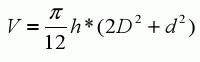where:
D is the large diameter of the barrel
d is the small diameter of the barrel
h is the height of the barrel
You may also optionally specify the material the barrel is constructed from to calculate the mass. The mass is calculated based on the volume of the barrel and the density of the material. The density of the material is derived from its specific gravity. Specific Gravities are available for a variety of metals, plastics and woods.
This calculator allows a user to specify English (inches, feet) or Metric (centimeters, meters) as possible units of measure for the diameters and height of the barrel. NOTE: All lengths are assumed to be of the same unit of measurement.

The Properties of a Cone Calculator is used to calculate the volume, face surface area, and total surface area of a cone, using the following... (Read More)
The Properties of a Cone Calculator is used to calculate the volume, face surface area, and total surface area of a cone, using the following equations: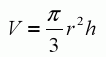where:
r is the radius of the cone's base
h is the height of the cone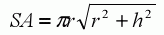where:
r is the radius of the cone's base
h is the height of the cone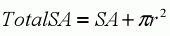where:
r is the radius of the cone's base
SA is the cone's face surface area
You may also optionally specify the material the cone is constructed from to calculate the mass. The mass is calculated based on the volume of the barrel and the density of the material. The density of the material is derived from its specific gravity. Specific Gravities are available for a variety of metals, plastics and woods.
This calculator allows a user to specify English (inches, feet) or Metric (centimeters, meters) as possible units of measure for the radius and height of the cone. NOTE: All lengths are assumed to be of the same unit of measurement.

The Properties of a Cylinder Calculator is used to calculate the volume, face surface area, and total surface area of a cylinder, using the following... (Read More)
The Properties of a Cylinder Calculator is used to calculate the volume, face surface area, and total surface area of a cylinder, using the following equations: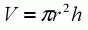where:
r is the radius of the cylinder
h is the height of the cylinder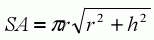where:
r is the radius of the cylinder
h is the height of the cylinder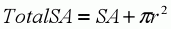where:
r is the radius of the cylinder
SA is the cylinder's face surface area
You may also optionally specify the material the cylinder is constructed from to calculate the mass. The mass is calculated based on the volume of the cylinder and the density of the material. The density of the material is derived from its specific gravity. Specific Gravities are available for a variety of metals, plastics and woods.
This calculator allows a user to specify English (inches, feet) or Metric (centimeters, meters) as possible units of measure for the radius and height of the cone. NOTE: All lengths are assumed to be of the same unit of measurement.

The Properties of a Hollow Cylinder Calculator is used to calculate the volume of a hollow cylinder, using the following equation: where: R is the... (Read More)
The Properties of a Hollow Cylinder Calculator is used to calculate the volume of a hollow cylinder, using the following equation: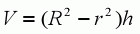where:
R is the outer radius of the cylinder
r is the inner radius of the cylinder
You may also optionally specify the material the cylinder is constructed from to calculate the mass. The mass is calculated based on the volume of the cylinder and the density of the material. The density of the material is derived from its specific gravity. Specific Gravities are available for a variety of metals, plastics and woods.
This calculator allows a user to specify English (inches, feet) or Metric (centimeters, meters) as possible units of measure for the radius and height of the cone. NOTE: All lengths are assumed to be of the same unit of measurement.

The Properties of a Sector of a Sphere Calculator is used to calculate the volume and surface area of a spherical sector. A spherical sector is a... (Read More)
The Properties of a Sector of a Sphere Calculator is used to calculate the volume and surface area of a spherical sector. A spherical sector is a solid of revolution enclosed by two radii from the center of a sphere. (Find out more at Wolfram Mathworld) The following equations are used to calculate the sector's volume and surface area: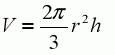where:
r is the radius of the sphere
h is the height of the spherical sector (the distance between the points where the two radii intersect the edge of the sphere)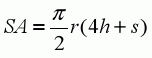where:
r is the radius of the sphere
h is the height of the spherical sector (the distance between the points where the two radii intersect the edge of the sphere)
s is the diameter of the circular base of the sector.
You may also optionally specify the material the sphere is constructed from to calculate the mass. The mass is calculated based on the volume of the sector of the sphere and the density of the material. The density of the material is derived from its specific gravity. Specific Gravities are available for a variety of metals, plastics and woods.
This calculator allows a user to specify English (inches, feet) or Metric (centimeters, meters) as possible units of measure for the radius and height of the cone. NOTE: All lengths are assumed to be of the same unit of measurement.

The Properties of a Sphere With Cylindrical Boring Calculator is used to calculate the volume and surface area of a sphere with cylindrical boring... (Read More)
The Properties of a Sphere With Cylindrical Boring Calculator is used to calculate the volume and surface area of a sphere with cylindrical boring using the following equations: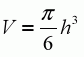where:
h is the height of the cylindrical boringwhere:
h is the height of the cylindrical boring
R is the radius of the sphere
r is the radius of the cylindrical boring
You may also optionally specify the material the sphere is constructed from to calculate the mass. The mass is calculated based on the volume of the sphere and the density of the material. The density of the material is derived from its specific gravity. Specific Gravities are available for a variety of metals, plastics and woods.
This calculator allows a user to specify English (inches, feet) or Metric (centimeters, meters) as possible units of measure for the radius and height of the cone. NOTE: All lengths are assumed to be of the same unit of measurement.

The Properties of a Torus Calculator is used to calculate the volume and surface area of a torus using the following equations: where: D is the... (Read More)
The Properties of a Torus Calculator is used to calculate the volume and surface area of a torus using the following equations: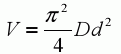where:
D is the large diameter of the torus
d is the small diameter of the torus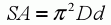where:
D is the large diameter of the torus
d is the small diameter of the torus
You may also optionally specify the material the torus is constructed from to calculate the mass. The mass is calculated based on the volume of the torus and the density of the material. The density of the material is derived from its specific gravity. Specific Gravities are available for a variety of metals, plastics and woods.
This calculator allows a user to specify English (inches, feet) or Metric (centimeters, meters) as possible units of measure for the radius and height of the cone. NOTE: All lengths are assumed to be of the same unit of measurement.

### Flow Control & Fluid Transfer

The Compressible Flow Pressure Loss Calculator is used determine the pressure loss for compressible, flowing gases. Calculations are based on gas... (Read More)
The Compressible Flow Pressure Loss Calculator is used determine the pressure loss for compressible, flowing gases. Calculations are based on gas properties such as molecular weight and specific heat ratio, and physical specifications for pipes, tubes, valves, and fittings. This calculator is designed for use with clean commercial steel pipes, galvanized iron pipes, drawn tubing, cast iron pipes, PVC pipes, fiberglass pipes, and asphalted cast iron pipes.

The Gas Density and Specific Volume Calculator calculates the density and specific volume of gas based on a modified version of the Ideal Gas Law:... (Read More)
The Gas Density and Specific Volume Calculator calculates the density and specific volume of gas based on a modified version of the Ideal Gas Law: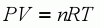where:
P is the absolute pressure of the gas,
V is the volume of the gas,
n is the number of moles of gas,
T is the absolute temperature of the gas,
R is the universal gas constant
The Ideal Gas Law assumes the existence of a gas with no volume and no interactions with other molecules. Therefore, the Compressibility Factor (Z) can be used for a slight alteration to the ideal gas law to account for real gas behavior. Therefore the equation used for these calculations is: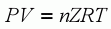where:
P is the absolute pressure of the gas,
V is the volume of the gas,
n is the number of moles of gas,
T is the absolute temperature of the gas,
R is the universal gas constant
Z is the gas compressibility factor
This equation is sometimes referred to as the Non-Ideal Gas Law
This calculator has been scaled to use English units for temperature and pressure. Molecular weights for acetylene, air, ammonia, argon, carbon dioxide, carbon monoxide, chlorine, hydrogen, natural gas, nitrogen, oxygen, propane and steam are all provided.

The Loss in Fluid Lines Calculator is used to determine pressure loss and head loss for standard-sized piping. Calculations are based on fluid... (Read More)
The Loss in Fluid Lines Calculator is used to determine pressure loss and head loss for standard-sized piping. Calculations are based on fluid properties such as viscosity and flow rate, and physical specifications for pipes, valves, and fittings. This calculator provides some typical viscosity and specific gravity values for gasoline, water, and SAE 30 motor oil. It also accounts for increases in pressure drop due to scale, dirt, and corrosion.

The Open Channel Flow Calculator is used to determine open-channel flow in circular pipes based on Manning's Equation: where: V is the cross-sectional... (Read More)
The Open Channel Flow Calculator is used to determine open-channel flow in circular pipes based on Manning's Equation: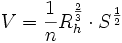where:
V is the cross-sectional average velocity (m/s)
n is the Manning coefficient of roughness
Rh is the hydraulic radius (m)
S is the slope of the water surface or the linear hydraulic head loss (m/m) (S = hf / L)
This calculator has been scaled to use English units. The Manning coefficient of roughness is built in for the following types of pipes: cast iron pipes in fair condition, riveted steel pipes, vitrified sewer pipes, concrete pipes, wood-stave pipes, planed-plank pipes, and smooth or corrugated semicircular metal flumes.

The Pressure Loss in Straight Gas Lines Calculator is used to determine the pressure loss from friction in straight pipes or tubes that transport... (Read More)
The Pressure Loss in Straight Gas Lines Calculator is used to determine the pressure loss from friction in straight pipes or tubes that transport gases. This calculator is useful for very small piping or tubing, and can handle flow through small channels. It includes inlet and outlet losses, but cannot accommodate losses due to fitting and valves. For standard-sized pipes, use the Loss in Flow Lines Calculator instead.

The Pressure Loss in Straight Liquid Lines Calculator is used to determine the pressure loss from friction in straight pipes or tubes that transport... (Read More)
The Pressure Loss in Straight Liquid Lines Calculator is used to determine the pressure loss from friction in straight pipes or tubes that transport liquids. This calculator is suitable for very small piping or tubing, and can handle flow through small channels. It includes inlet and outlet losses, but cannot accommodate losses due to fittings and valves. For standard-sized pipes, use the Loss in Fluid Lines Calculator instead.

The Self-Venting Flow Line Calculator is used to size self-venting, vertical pipes and tubes, or to size pipes and tubes for side-outlet applications.... (Read More)
The Self-Venting Flow Line Calculator is used to size self-venting, vertical pipes and tubes, or to size pipes and tubes for side-outlet applications. This calculator uses three pipe schedules: SCH 40, SCH 80 and SCH 160. The actual and nominal line sizes required for self-venting flow are measured in inches.

### Tanks & Vessels

The Drain Time for Vertical Tanks Calculator is used to determine the time to drain a vertical vessel between any two liquid levels. The calculator is... (Read More)
The Drain Time for Vertical Tanks Calculator is used to determine the time to drain a vertical vessel between any two liquid levels. The calculator is applicable to vessels with flat, elliptical, or hemispherical bottom heads.

The Volume of Partially-Filled Vertical Vessels Calculator is used to determine volumes of partially filled vertical vessels and tanks. The calculator... (Read More)
The Volume of Partially-Filled Vertical Vessels Calculator is used to determine volumes of partially filled vertical vessels and tanks. The calculator is applicable to vessels with flat, elliptical, hemispherical or conical heads.

### Electrical Calculators

Capacitors are electrical devices that are used to store electrical energy. A typical capacitor consists of two fixed conducting plates (electrodes)... (Read More)
Capacitors are electrical devices that are used to store electrical energy. A typical capacitor consists of two fixed conducting plates (electrodes) which face each other and are separated by a distance (d) An insulating material (such as air) fills the gap between the two plates. This insulating material is called the dielectric.
When a potential difference or voltage (V, volts) is applied across the two plates, an amount of opposite and equal charge (Q, coulombs) is stored in each electrode. Capacitance (F, farads) is the charge per unit volt that a capacitor can store, Because a farad is an extremely large unit, capacitances are measured normally in micro farads ( F), nano farads (nF), or pico farads (pF).
Because a capacitor’s plates are fixed, an electric field will develop. As a consequence of this, a voltage develops across the plates. As more charges accumulate, the voltage increases. The voltage of the applied potential is the maximum voltage that can appear across the capacitor. The amount of charge that can be stored in the plates is a function of the plate areas (A, in meters), the permittivity of the dielectric ( , in farads per meter), and the separation of the plates (d). The energy stored in the capacitor (E, joules), due to the accumulation of charges, is a measure of the amount of work needed to develop the voltage across the plates.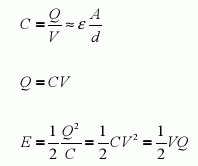Ohm's Law defines the relationship between voltage (V), current (I), power (P) and resistance (R) in electrical circuits.... (Read More)
Ohm's Law defines the relationship between voltage (V), current (I), power (P) and resistance (R) in electrical circuits. It was discovered in 1827 by Georg Ohm (1789 – 1854), a German mathematician and physicist who, as a high school teacher, decided to study Alessandro Volta's new invention: the electromechanical cell (the precursor of the battery).
The relationships between the four important parameters of circuits are as follows:
1. Voltage (V, in volts) calculations: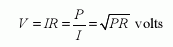2. Current (I, in amps) calculations: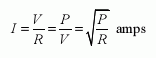3. Power (P, in watts) calculations: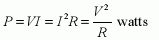4. Resistance (R, in Ω) calculations: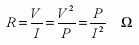To use this calculator, enter a value for any two of the following: voltage, current, resistance, and power.

Voltage dividers are electric circuits used to scale down a voltage by a given fraction. The output voltage of the circuit is proportional to the... (Read More)
Voltage dividers are electric circuits used to scale down a voltage by a given fraction. The output voltage of the circuit is proportional to the input voltage (source voltage), but smaller. The circuit divides the source voltage into two parts. Generally, the output voltage of the divider is used to drive another circuit (load). Alternatively, this output can be used as a reference voltage.
The output voltage for this circuit can be found with the following equation: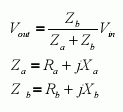This calculator assumes there is no load on this circuit. If you need to calculate the voltage out for a circuit without a load then use the Voltage Divider Calculator For AC Circuits With Load

Voltage dividers are electric circuits used to scale down a voltage by a given fraction. The output voltage of the circuit is proportional to the... (Read More)
Voltage dividers are electric circuits used to scale down a voltage by a given fraction. The output voltage of the circuit is proportional to the input voltage (source voltage), but smaller. The circuit divides the source voltage into two parts. Generally, the output voltage of the divider is used to drive another circuit (load). Alternatively, this output can be used as a reference voltage.
The output voltage for this circuit can be found with the following equation:This calculator assumes there is a load on this circuit. If you need to calculate the voltage out for a circuit without a load then use the Voltage Divider Calculator For AC Circuits

Voltage dividers are electric circuits used to scale down a voltage by a given fraction. The output voltage of the circuit is proportional to the... (Read More)
Voltage dividers are electric circuits used to scale down a voltage by a given fraction. The output voltage of the circuit is proportional to the input voltage (source voltage), but smaller. The circuit divides the source voltage into two parts. Generally, the output voltage of the divider is used to drive another circuit (load). Alternatively, this output can be used as a reference voltage.
The output voltage for this circuit can be found with the following equation: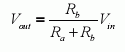This calculator assumes there is no load on this circuit. If you need to calculate the voltage out for a circuit with load then use the Voltage Divider Calculator For DC Circuits With Load

Voltage dividers are electric circuits used to scale down a voltage by a given fraction. The output voltage of the circuit is proportional to the... (Read More)
Voltage dividers are electric circuits used to scale down a voltage by a given fraction. The output voltage of the circuit is proportional to the input voltage (source voltage), but smaller. The circuit divides the source voltage into two parts. Generally, the output voltage of the divider is used to drive another circuit (load). Alternatively, this output can be used as a reference voltage.
The output voltage for this circuit can be found with the following equation:This calculator assumes there is a load on this circuit. If you need to calculate the voltage out for a circuit without a load then use the Voltage Divider Calculator For DC Circuits

In electrical systems, impedance mismatches cause some of the transmitted energy to be reflected back to its source. The frequency of the signal... (Read More)
In electrical systems, impedance mismatches cause some of the transmitted energy to be reflected back to its source. The frequency of the signal determines the amount of energy that is reflected. Voltage Standing Wave Ratio (VSWR), a unitless ratio ranging from 1 to infinity, expresses the amount of reflected energy at the input or output of the device. A value of 1 indicates that all of the energy passes through.
VSWR is defined as the maximum voltage of the standing wave (Emax) to the minimum voltage of the standing wave (Emin). Any other value indicates that a portion of the energy is reflected. There is an equivalent definition for VSWR, which can also be expressed in terms of the Return Loss (RL). The return loss is the ratio of the power of the wave reflected to that of the incident wave. It is generally expressed in decibels (dB).
The Reflection Coefficient (ρ), a figure of merit related to VSWR and to the return loss, is defined as the ratio of the reflected voltage to the incident voltage. Finally, another measurement of mismatching of impedances is the Mismatch Loss (ML). This is a measure of how much the power of the transmitted wave is attenuated due to reflection. These four parameters are related through the following formulas: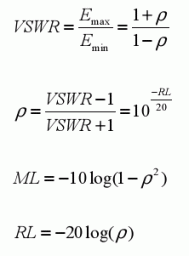To use this calculator enter a value for any one of the following: VSWR, Reflection Coefficient, Return Loss